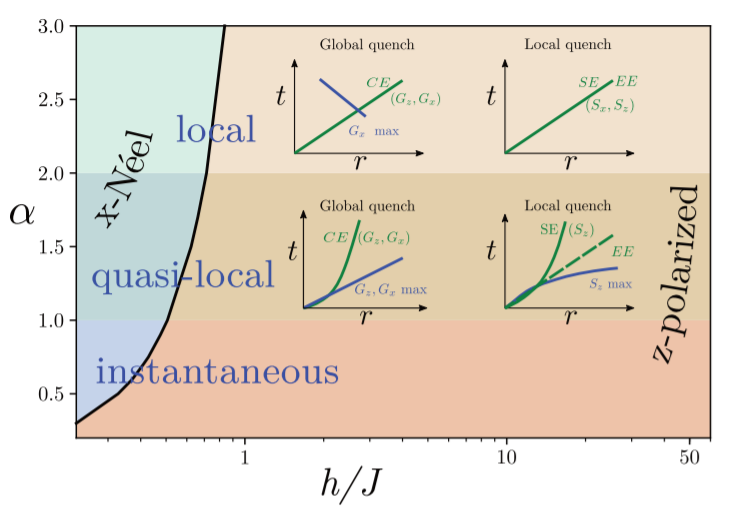Date

A research group led by Jan Schneider wich counts with the participation of Luca Tagliacozzo of the Institute of Cosmos Sciences of the University of Barcelona (ICCUB) published a paper in the March 2021 issue of Physical Review Research where the spreading of correlations and entanglement in the long-range transverse Ising chain is analyzed.

In the paper, the authors discuss the paradigmatic model for the long-range transverse Ising chain. This model is characterized by interactions that fall off with the distance as 1/Rα. Such long-range interactions can now be emulated in quantum simulations and this opens a wide range of possibilities for their study.

Depending on the value of ⍺, one can distinguish three separate regimes: the local regime (α>2) which is short-range, the instantaneous regime (0<α<1) which is long-range and then the intermediate or quasilocal regime (1<α<2).

On one hand, in the local regime all signals propagate causally and we find the presence of a Lieb-Robinson bound that implies that correlations decay exponentially beyond the limit set by the system’s maximal group velocity. On the other hand, in the instantaneous regime  causality completely breaks down. This is shown by the absence of Lieb-Robinson bounds and the vanishing of the characteristic dynamical time scale in the thermodynamic limit. The lesser known and therefore more interesting regime corresponds to the intermediate or quasilocal regime. The aim of the paper is, thus, to numerically characterize the spreading of quantum correlations in the long-range transverse Ising chain in this intermediate regime.

Theoretically, the known bounds present in the quasilocal regime allow for superballistic propagations but numerical simulations point toward a significantly slower propagation. Thus, the authors found that a weak form of causality emerges in this regime characterized by generic algebraic scaling forms such as:

t ∼ R𝛽

Depending on the value of the exponent 𝛽 one can distinguish three types of propagation: sub-ballistic propagation (𝛽>1), ballistic propagation (𝛽=1) and superballistic propagation (𝛽<1).

The authors proceed then to study the scaling laws of the spin-correlation (CE) and local spin (SE) causal edges and find that the CE and SE are both sub-ballistic (𝛽CE = 𝛽SE > 1) but the local maxima close to the causal edge propagates ballistically in the case of spin-correlations (𝛽mCE = 1) and superballistcally in the case of local spins (𝛽mSE < 1).

Comparing the fitted dynamical exponents 𝛽mCE and 𝛽CE to the predictions of the Linear Spin Wave Theory (LSWT), one finds excellent agreement.

So, in the case of spin-correlations, while the maxima spread ballistically, the correlation edge is sub-ballistic. This confirms the emergence of a weak, slower-than-ballistic form of causality in this quasilocal regime.Fig. 1. Phase diagram and dynamical properties of the LRTI chain in the (h/J, α) plane. The x-Néel phase (shades of blue) is separated from the z-polarized phase (shades of orange) by a critical line in black. The three dynamical regimes are shaded differently. As shown in the insets, the local and quasilocal regimes are characterized by distinct algebraic scaling laws for the correlation and spin edges (CE and SE, respectively), their maxima in space-time (max), and the entanglement edge (EE), after global or local quench. The different behaviors of these observables in different regions of the phase diagram provide clear, experimentally accessible, signatures of the local and quasilocal regimes.

This result sheds light on the propagation of information in long-range interacting lattice models and paves the way to future experiments as well as several quantum information transport devices. We are excited to see how this area unravels itself and where it is headed in the near future.